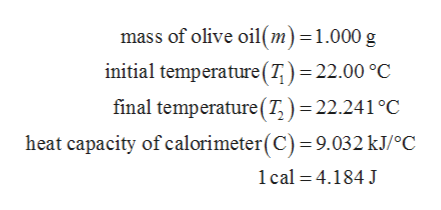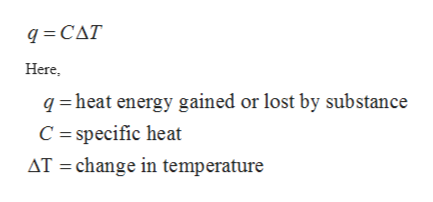# When 1.000 g of olive oil is completely burned in pure oxygen in a bomb calorimeter, the temperature of the water bath increases from 22.000ºC to 22.241ºC.a. How many dietary calories(Cal) (1 Cal = 1 kcal) are in olive oil, per gram? The heat capacity of the calorimeter is 9.032 kJ/ºC and 1cal. = 4.184J. b. Olive oil is pure glyceryl trioleate, C57H104O6. The equation of the combustion of thissubstance is :C57 H104O6(l) + 80 O2(g)→57 CO2(g) + 52 H2O(l) What is the heat of combustion of glyceryl trioleate in kJ per mol?

Question
362 views
When 1.000 g of olive oil is completely burned in pure oxygen in a bomb calorimeter, the temperature of the water bath increases from 22.000ºC to 22.241ºC.
a. How many dietary calories(Cal) (1 Cal = 1 kcal) are in olive oil, per gram? The heat capacity of the calorimeter is 9.032 kJ/ºC and 1cal. = 4.184J.

b. Olive oil is pure glyceryl trioleate, C57H104O6. The equation of the combustion of thissubstance is :
C57 H104O6(l) + 80 O2(g)→57 CO2(g) + 52 H2O(l)

What is the heat of combustion of glyceryl trioleate in kJ per mol?
check_circle

Step 1

The values are given as follows:help_outlineImage Transcriptionclosemass of olive oil(m) = 1.000 g initial temperature (7) = 22.00 °C final temperature (T) 22.241°C heat capacity of calorimeter(C) = 9.032 kJ/°C 1cal 4.184 J fullscreen
Step 2

The number of dietary calories can be calculated with the help of heat capacity formula as follows:help_outlineImage Transcriptioncloseq CAT Here qheat energy gained or lost by substance C = specific heat AT change in temperature fullscreen
Step 3

Substitute the given values into the above equation in order t...

### Want to see the full answer?

See Solution

#### Want to see this answer and more?

Solutions are written by subject experts who are available 24/7. Questions are typically answered within 1 hour.*

See Solution
*Response times may vary by subject and question.
Tagged in

### Inorganic Chemistry